Total of questions: 232

 First steps (3 questions) Basic Settings (19 questions) Cells (4 questions)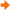Formulas and Functions (34 questions) Formatting (11 questions) Charts (21 questions) Special functions 1 (20 questions) Special functions 2 (19 questions) Images (3 questions) Data (30 questions) Sortings, Filters, Macros, Templates (17 questions) DIPLOMA level questions (6 questions) MS Excel Expert 2016, 2013, 2010, 2007, 2003 (BENCHMARK) (30 questions) MS Excel Expert 2016, 2013, 2010, 2007, 2003 (ASSESSMENT) (15 questions)

 1109 Enter the appropriate function in the cell C14 so as to calculate the maximum sales figure for January (cell range C5:C8).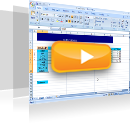1110 In the cell C15 enter a function which returns the word DELLA, if the content of the cell C5 is equal to the content of the cell C14; otherwise the formula returns the word other.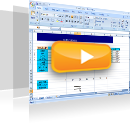1200 In the cell A1 enter a function that counts non blank cells for the cell range A3:A15000. Also, in the cell C116 enter a function that returns the number of cells that contain numbers for the cell range C3:C110 and in the cell C117 enter another function to return non blank cells for the same cell range.1201 In the cell E116 enter a function that calculates the average cost of the products displayed in the cell range Å3:Å110.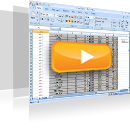1202 In the cell E116 enter a function that calculates the minimum cost for the cell range E3:E110 and in the cell E117 another function that returns the maximum cost for the same cell range.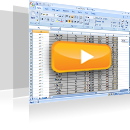1217 Enter the appropriate function in the cell B1, so that if the value in the cell (A1) is higher than 5000, the function will return 1. If the value in the cell A1 is less than or equal to 5000, the function will return 0. Reproduce the function up to the cell B10.1218 Insert the appropriate function in the cell E1, so that if the value in the cell A1 is higher than the value in the cell B1, the function will return the value of the cell C1; otherwise the function will return the value of the cell D1. Reproduce this function up to the cell E10.1308 Enter in the cell E116 a function to calculate the minimum value of the cell range Å3:Å110.1310 Insert in the cell C116 a function to count the total of cells containing numbers within the cell range C3:C110.1311 Insert a function in the cell A1 to count non blank cells within the cell range A3:A15000.1528 In the cell B1 of the Total worksheet insert the appropriate function to sum up the cells within the cell range A1:A10 from the FF1 worksheet through to the FF12 worksheet. Insert in the cell B2 a function that returns the average of the respective cell range, in cell B3 for the minimum, in cell B4 for the maximum and in cell B5 for the total of cells displaying numbers, following the same pattern.1542 Insert the appropriate function in the cell A1 to sum up values greater than or equal to 5000 in the cell range A2:A100. Insert the appropriate function in the cell B1 to sum up the cells, the corresponding cells of which in column A display a value greater than or equal to 5000, in the cell range B2:B100.1543 Insert the appropriate function in the cell range B1:B50 that rounds the values of column A to 4 decimal places. Insert the appropriate function in the cell range C1:C50 that rounds the values of column A to 2 decimal places. Insert the appropriate function in the cell range D1:D50 that rounds the values of column A to the left of the decimal point.1544 Insert the appropriate function in the cell A1 that returns how many times the value CPU is displayed in the cell range A3:A50. Insert the appropriate function in the cell B1 that returns the count of cells containing values less than or equal to 20 in the cell range B3:B50.1545 Insert the appropriate function in the cell A2 that changes the format on the contents of the cell A1 to title case. Insert the appropriate function in the cell A3 that changes the format on the contents of the cell A1 to uppercase. Insert the appropriate function in the cell A4 that changes the format on the contents of the cell A1 to lowercase.1546 In the cell A5 insert the appropriate function that joins the text of the cells A1, A2, A3, A4. In the cell A6 insert the appropriate formula (not a function) that joins the text of the cells A1, A2, A3, A4.1671 Use the appropriate function in the cell G12 to calculate the average of the values displayed in the cell range G5:G10.1679 Use the appropriate function in the cell J4 to calculate the average number of items sold in April.1706 Use the appropriate function in the cell C8 to display the value 198, if the cell C2 value is higher than or equal to 3000. Otherwise, the function returns the value 0.198.1711 Use the appropriate function in the cell J4 to display the lowest value in the cell range D5:D10.1712 Navigate to the cell D12 and calculate the maximum value of the cell range D5:G10.1714 Navigate to the cell J4 and insert there the appropriate function to display the maximum value of the cell range F5:F10.1718 Navigate to the cell G13 and insert there the appropriate function to display the sum of the values appearing in the cell range G4:G10. Then, save changes.1731 Navigate to the cell A8 and use the appropriate function to calculate how many cells are displaying numbers within the cell range A1:A6.1750 Navigate to the cell H17 of the active worksheet and use the appropriate function to display the total of the cell range H6:H9.1758 Navigate to the cell E11 of the SALES worksheet and insert there the appropriate function to display the sum of the values of the cell range E6:E9.1768 Navigate to the cell C14 of the active worksheet and insert there the appropriate function to display the average of the values of the cell range D6:D9.1844 Use the appropriate function in the cell E11, to return the day of the date displayed in the cell E4.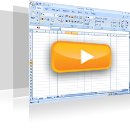1845 Use the appropriate function in the cell E14 to round the contents of the cell E11 to two decimal places.1861 Open the Report.xltx template located in the IL-ates\Excel folder on your Desktop. Correct the formulas entered in cells C32 and D32 and save the template as Report2.xltx to the same folder.1874 Enter the appropriate function in the cell range D5:D16 to return the Expenses status as follows: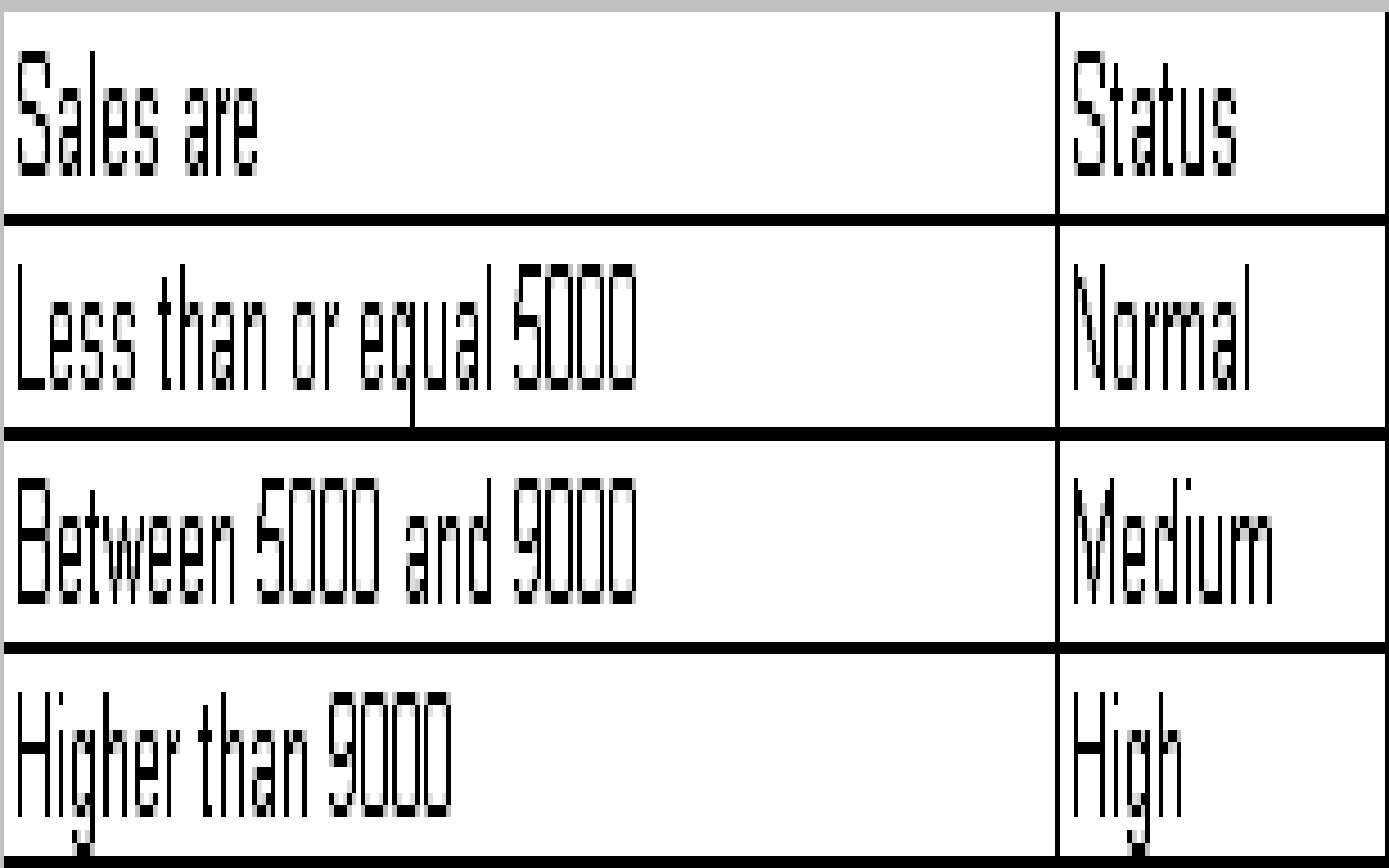1880 Use the proper function in the cell B1 to count the blank cells in the cell range A1:A400.1923 Delete the name of the cell range insert.1927 Calculate the sum of the cell range called sumArea in the cell A1 using the appropriate function.